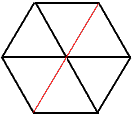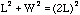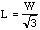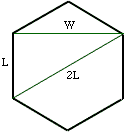Date: Tue, 22 Sep 1998 10:31:32 -0600 Sender: CURTIS CONSTRUCTION Subject: hexagon Could someone calculate this for me? If a hexagon is 38 feet 8 inches across, how wide would each of the six sides be? Thank you, Lee Hi Lee,
 No doubt we are looking at a regular hexagon (which is composed of 6 equilateral triangles sharing one vertex at the centre of the hexagon).The diagonal of a regular hexagon, coloured red in the diagram, is twice the side length, so one possible answer to the question is that the sides are 19'4" long.If, however, the width refers to the distance between parallel sides, labeled W in the diagram, consider the triangle shown with sides of length W, L and 2L. This is a right angled triangle so the theorem of Pythagoras insures thatSolving this equation for L givesIn your case W=38.666... feet so L is approximately 38.666/1.732  = 22.3242 feet which is approximately 22 feet 4 inches. Cheers, Chris and HarleyGo to Math Central

To return to the previous page use your browser's back button.